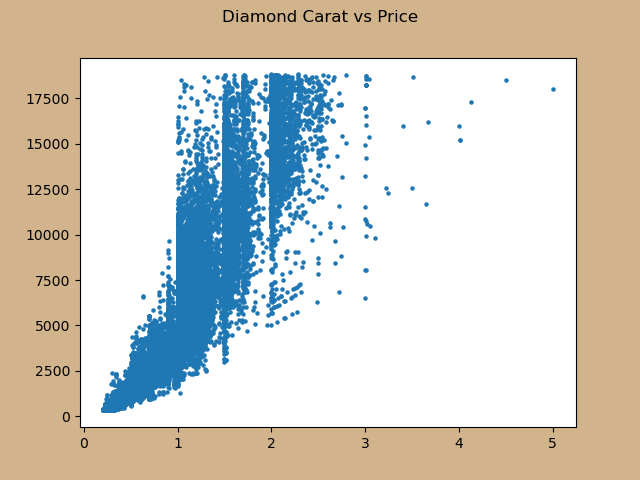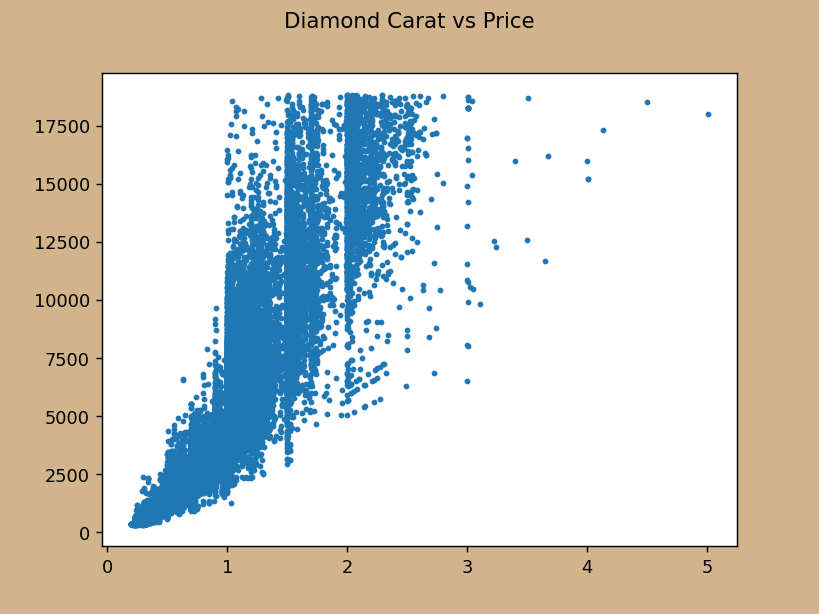Dec 09, 2021

# Why Matplotlib Figure Inches Don't Match Your Screen Inches and How to Fix it¶

If you've worked with the matplotlib data visualization library before, then you'll be familiar with the term figsize, which is measured in figure inches. These default to 6.4 inches in width by 4.8 inches in height. But, if you actually measured the physical screen inches on your monitor, you're likely to get different numbers. This post details why this mismatch exists and how to change the settings of your plot so that the matplotlib figure inches match the physical screen inches exactly.

### Create a plot¶

We begin by reading in the diamonds dataset and create a scatter plot of carat vs price.

In :
```import pandas as pd
```
Out:
carat cut color clarity depth table price x y z
0 0.23 Ideal E SI2 61.5 55.0 326 3.95 3.98 2.43
1 0.21 Premium E SI1 59.8 61.0 326 3.89 3.84 2.31
2 0.23 Good E VS1 56.9 65.0 327 4.05 4.07 2.31

Jupyter Notebooks automatically crop some of the figure so it tightly wraps around the outer elements. To show the entire figure we need to run the following command.

In :
```%config InlineBackend.print_figure_kwargs = {'bbox_inches': None}
```

For clarity, the `figsize` is set to `(6.4, 4.8)`, though this is unnecessary as that is the default value.

In :
```import matplotlib.pyplot as plt
fig, ax = plt.subplots(figsize=(6.4, 4.8), facecolor='tan')
ax.scatter(x='carat', y='price', data=diamonds, s=5)
fig.suptitle('Diamond Carat vs Price');
```### Measuring the physical screen inches¶

You can use a physical ruler or an application native to your machine to find the physical screen inches. I used an application called Free Ruler on my Mac and captured the image below. The actual physical screen inches of the figure are about 5 x 3.75 or under 80% of the matplotlib figure size of 6.4 x 4.8.### DPI Mismatch¶

The reason for this difference is a mismatch between the matplotlib figure DPI (dots per inch) and your screen's DPI. By default, Jupyter Notebooks set the DPI for matplotlib figures to 100, which we verify below.

In :
```fig.dpi
```
Out:
`100.0`

Multiplying the DPI by the figure inches provides the dimensions of the figure in pixels. This makes our current figure 640 pixels by 480 pixels. Most modern monitors have a higher DPI than than 100, which is the reason the figures appear as less physical screen inches. For example, if your monitor has a DPI of 160, then the physical screen inches would be 4 inches by 3 inches (640 / 160 and 480 / 160).

Getting your screen's DPI is a little tricky. The settings for my Mac show the screen as 3456 by 2234 pixels and 16.125 inches in diagonal, but do not display the DPI itself. Also, the pixel dimensions can be scaled down below their stated values. I used What is my Screen Resolution to get my specific pixel dimensions, which are really 1728 by 1117.

### Use function to get DPI¶

We still do not have our DPI, but can use the Pythagorean theorem to calculate them. The following function accepts the width and height as pixels and diagonal in inches and returns the DPI.

In :
```def find_dpi(w, h, d):
"""
w : width in pixels
h : height in pixels
d : diagonal in inches
"""
w_inches = (d ** 2 / (1 + h ** 2 / w ** 2)) ** 0.5
return round(w / w_inches)

find_dpi(1728, 1117, 16.125)
```
Out:
`128`

My monitor's DPI is 128. Let's divide the current pixel dimensions by this DPI to calculate physical screen inches and determine if it matches the measurement from Free Ruler above.

In :
```640 / 128, 480 / 128
```
Out:
`(5.0, 3.75)`

### Setting the figure DPI¶

Set the DPI upon figure creation by setting the `dpi` parameter.

In :
```fig, ax = plt.subplots(figsize=(6.4, 4.8), facecolor='tan', dpi=128)
ax.scatter(x='carat', y='price', data=diamonds, s=5)
fig.suptitle('Diamond Carat vs Price');
```### Physical screen inches should now be the same as the matplotlib figure inches¶

We verify with Free Ruler that the physical screen inches are the same as the matplotlib figure inches, 6.4 x 4.8.## Use this DPI for all your figures¶

Continue to set the `dpi` parameter to your monitor's DPI to have matplotlib figure inches match the physical screen inches. Note, that if you create a figure larger than viewable area in a notebook (30 x 15 for example), it will be scaled down to fit inside of a notebook cell and will not match the physical screen inches.

### Master Data Analysis with Python¶

If you enjoyed this tip, then check out my comprehensive book Master Data Analysis with Python that teaches you how to become an expert at using Python and Pandas to do data analysis.

Close